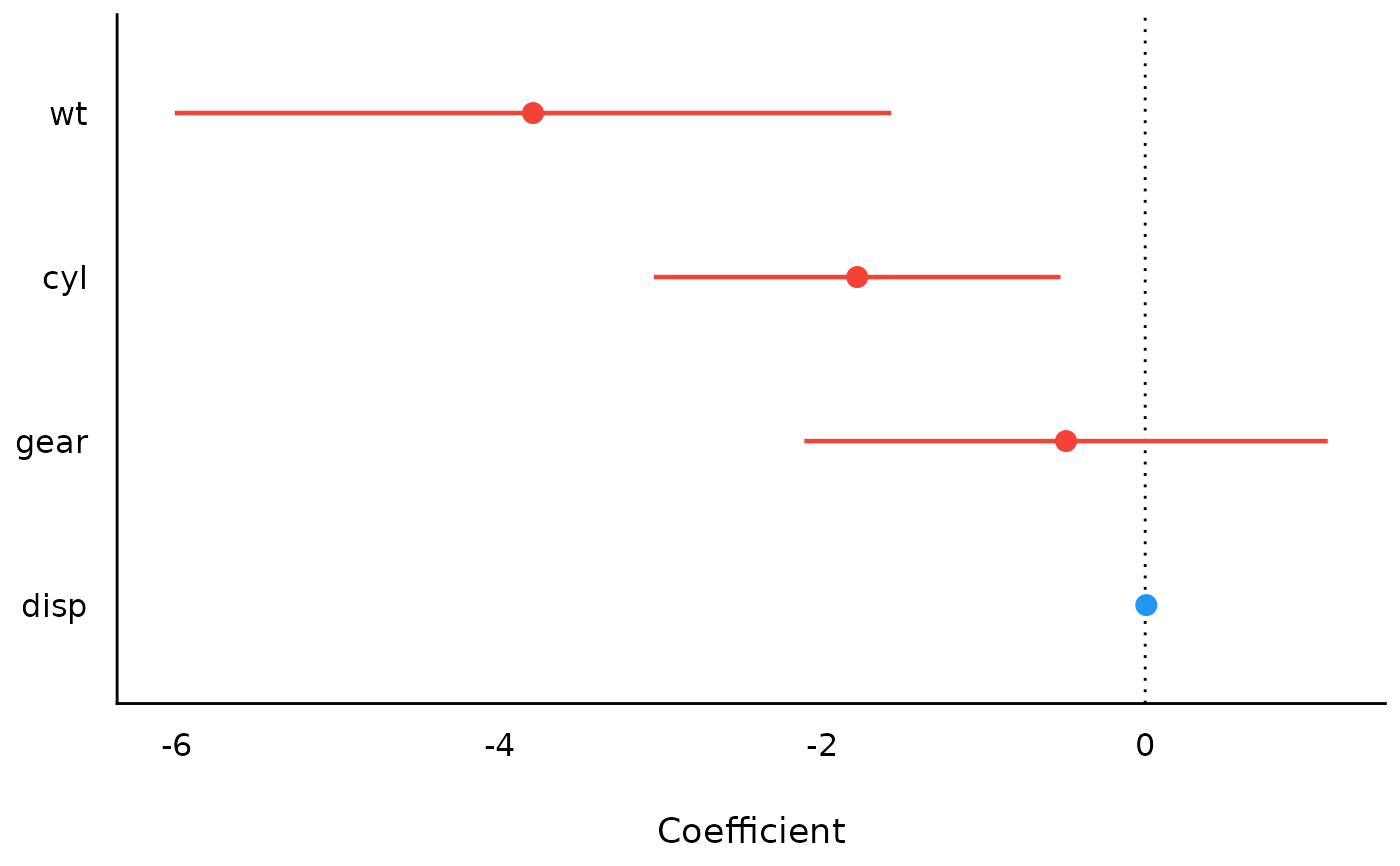The plot() method for the parameters::model_parameters() function.

## Usage

# S3 method for see_parameters_model
plot(
x,
show_intercept = FALSE,
size_point = 0.8,
size_text = NA,
sort = NULL,
n_columns = NULL,
type = c("forest", "funnel"),
weight_points = TRUE,
show_labels = FALSE,
show_estimate = TRUE,
show_interval = TRUE,
show_density = FALSE,
log_scale = FALSE,
...
)

# S3 method for see_parameters_sem
plot(
x,
data = NULL,
type = component,
threshold_coefficient = NULL,
threshold_p = NULL,
ci = TRUE,
size_point = 22,
...
)

## Arguments

x

An object.

show_intercept

Logical, if TRUE, the intercept-parameter is included in the plot. By default, it is hidden because in many cases the intercept-parameter has a posterior distribution on a very different location, so density curves of posterior distributions for other parameters are hardly visible.

size_point

Numeric specifying size of point-geoms.

size_text

Numeric value specifying size of text labels.

sort

The behavior of this argument depends on the plotting contexts.

• Plotting model parameters: If NULL, coefficients are plotted in the order as they appear in the summary. Setting sort = "ascending" or sort = "descending" sorts coefficients in ascending or descending order, respectively. Setting sort = TRUE is the same as sort = "ascending".

• Plotting Bayes factors: Sort pie-slices by posterior probability (descending)?

n_columns

For models with multiple components (like fixed and random, count and zero-inflated), defines the number of columns for the panel-layout. If NULL, a single, integrated plot is shown.

type

Character indicating the type of plot. Only applies for model parameters from meta-analysis objects (e.g. metafor).

weight_points

Logical. If TRUE, for meta-analysis objects, point size will be adjusted according to the study-weights.

show_labels

Logical. If TRUE, text labels are displayed.

show_estimate

Should the point estimate of each parameter be shown? (default: TRUE)

show_interval

Should the compatibility interval(s) of each parameter be shown? (default: TRUE)

show_density

Should the compatibility density (i.e., posterior, bootstrap, or confidence density) of each parameter be shown? (default: FALSE)

log_scale

Should exponentiated coefficients (e.g., odds-ratios) be plotted on a log scale? (default: FALSE)

...

Arguments passed to or from other methods.

data

The original data used to create this object. Can be a statistical model.

component

Character indicating which component of the model should be plotted.

threshold_coefficient

Numeric, threshold at which value coefficients will be displayed.

threshold_p

Numeric, threshold at which value p-values will be displayed.

ci

Logical, whether confidence intervals should be added to the plot.

## Value

A ggplot2-object.

## Examples

library(parameters)
m <- lm(mpg ~ wt + cyl + gear + disp, data = mtcars)
result <- model_parameters(m)
result
#> Parameter   | Coefficient |   SE |         95% CI | t(27) |      p
#> ------------------------------------------------------------------
#> (Intercept) |       43.54 | 4.86 | [33.57, 53.51] |  8.96 | < .001
#> wt          |       -3.79 | 1.08 | [-6.01, -1.57] | -3.51 | 0.002
#> cyl         |       -1.78 | 0.61 | [-3.04, -0.52] | -2.91 | 0.007
#> gear        |       -0.49 | 0.79 | [-2.11,  1.13] | -0.62 | 0.540
#> disp        |    6.94e-03 | 0.01 | [-0.02,  0.03] |  0.58 | 0.568
#>
#> Uncertainty intervals (equal-tailed) and p-values (two-tailed) computed
#>   using a Wald t-distribution approximation.
plot(result)